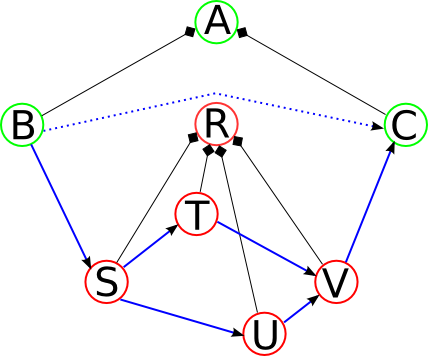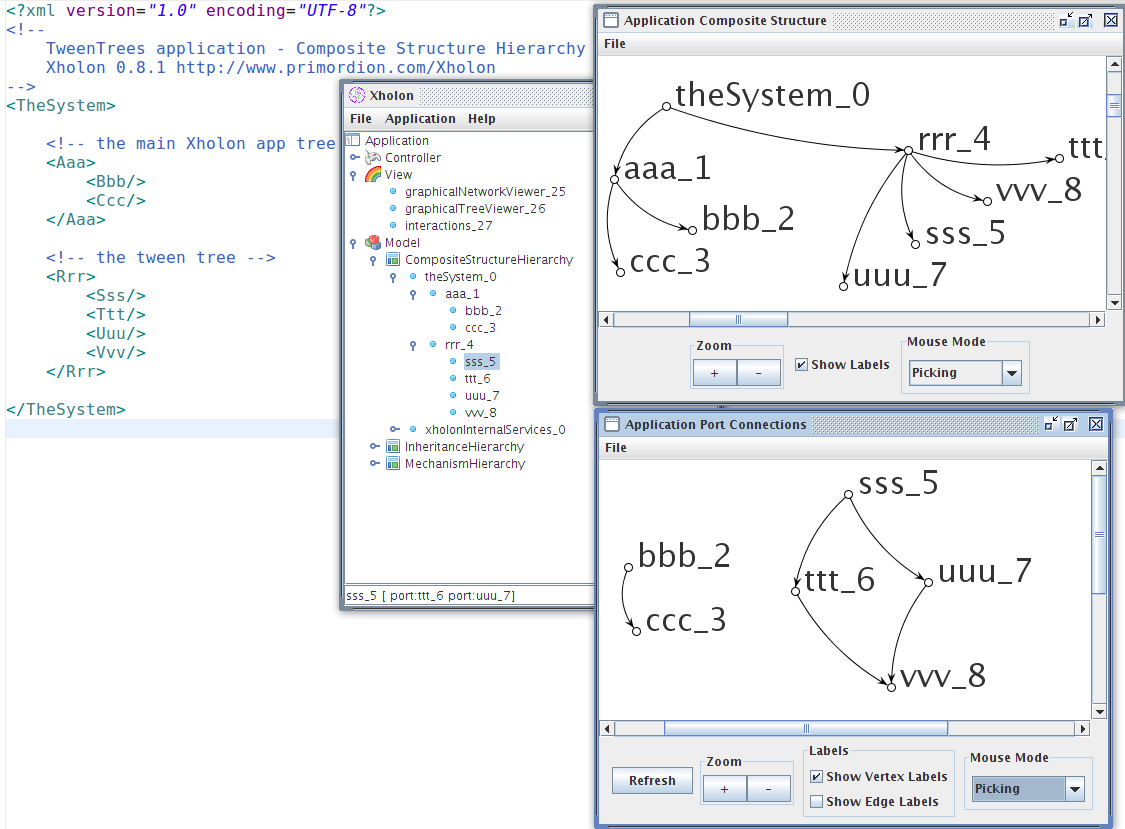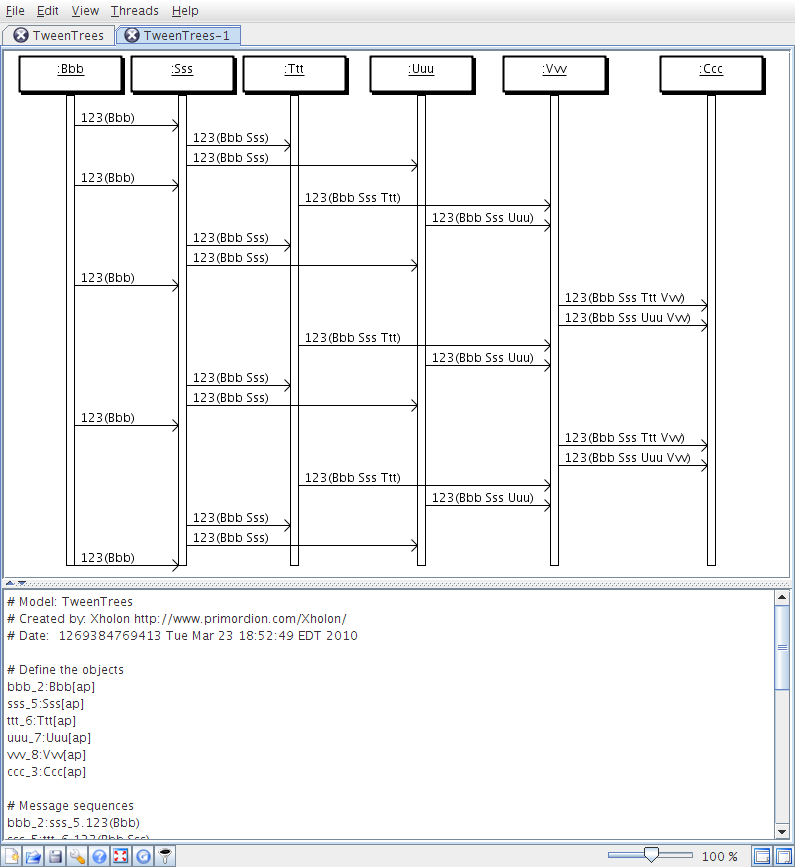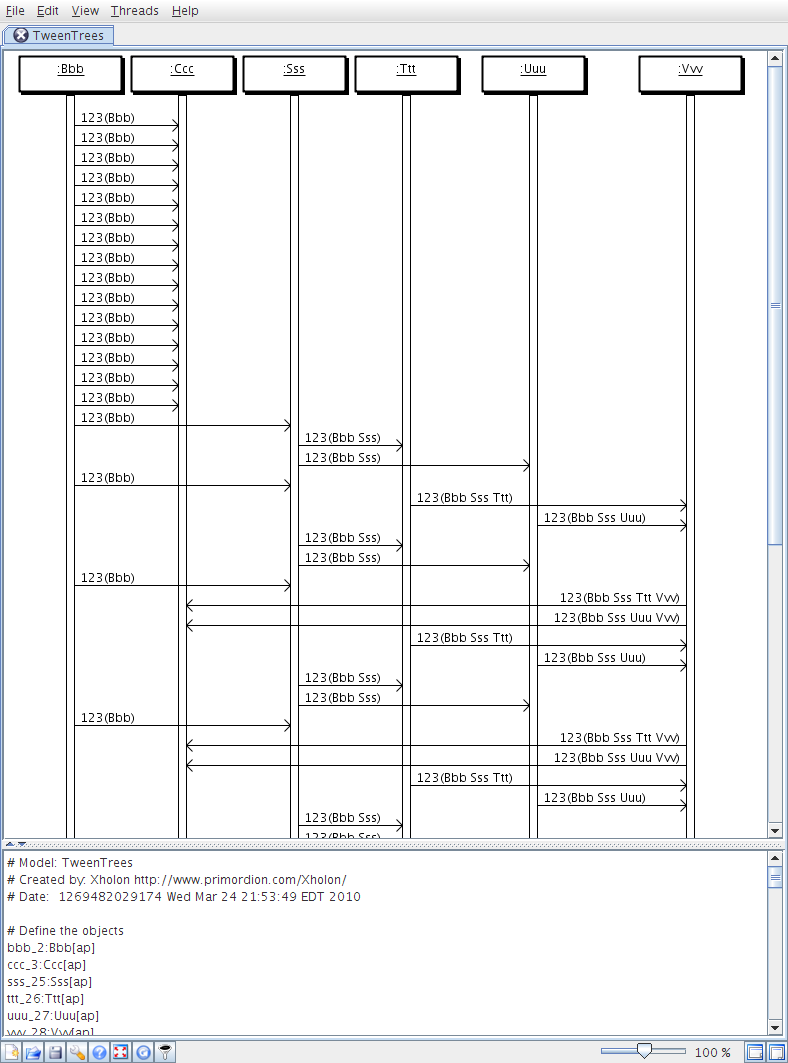### Tween Trees

Ken Webb 2010-03-26T13:23:04Z

In Xholon, a tween tree is a subtree that is not part of the main application tree. Instead, it is typically located along a connection between two other nodes that are part of the main Xholon tree.

The idea for tween trees originated while reading a blog entry by Ralf Westphal.

The Xholon way of dealing with a question like this is to build and run a sample application, and iteratively add the missing functionality to the Xholon core. To me, the challenge is always how to solve a problem by creating and running some software. And it's also important in Xholon that the solution be applicable to any other Xholon application. For example, it should be possible at runtime to select any edge in any application, and add some new structure to the edge. This might be a node or subtree able to monitor, redirect, or modify messages exchanged between the two vertices that share the edge. A good way to start exploring this would be to write a Xholon script, complete with a new subtree, and paste this into a running Xholon application such as the simple HelloWorld.

The following diagram shows a normal Xholon application tree, and a Tween Tree.Nodes A, B, and C belong to the main Xholon app tree. Nodes R, S, T, U, and V belong to the tween tree. A is the root of the main tree. R is the root of the tween tree. B and C are children of A. S, T, U, and V are children of R. The dashed connection from B to C is the original connection between these two nodes. The new connection between B and C is through the tween tree, from B to S to (T or U) to V to C.

The most important thing to note is that R is not a child of A.

I've constructed a sample Xholon application called TweenTrees. The initial implementation, without tween trees, is as follows.The Rrr node is the root of the tween tree. In a refinement of the implementation, it configures things as follows.

```public void postConfigure()
{
switch(xhc.getId()) {
case RrrCE:
/*
* At runtime, Rrr will be responsible for making itself and its subtree into a tween tree.
* It will:
* - disconnect B and C,
* - connect B to S,
* - connect V to C,
* - remove itself as a child of TheSystem and thus becoming a true tween tree.
*/
IXholon bbb = getXPath().evaluate("ancestor::TheSystem/Aaa/Bbb", this);
IXholon bbbTarget = bbb.getPort(0);
IXholon sss = getXPath().evaluate("./Sss", this);
IXholon vvv = getXPath().evaluate("./Vvv", this);
((XholonWithPorts)bbb).setPort(0, sss);
((XholonWithPorts)vvv).setPort(0, bbbTarget);
removeChild();
break;
default:
break;
}
super.postConfigure();
}
```

The tween tree nodes (Rrr, etc.) are now no longer visible in the Xholon GUI.But the tween tree nodes can still be discovered at runtime if Bbb sends messages out its port.

```port.sendMessage(123, getName("^^C^^^"), this);
```

The tween tree nodes can forward received messages, and add their own names to the message data.

```for (int i = 0; i < port.length; i++) {
if (port[i] != null) {
port[i].sendMessage(msg.getSignal(), msg.getData() + " " + getName("^^C^^^"), this); //msg.getSender());
}
}
```

After a few messages have worked their way through the tween tree from Bbb to Ccc, Xholon can generate a sequence diagram and send it to the Quick Sequence Diagram Editor (sdedit).So the initial test works. Xholon can support tween trees without the need for any changes to the framework itself.

### Using a runtime script

The next thing to try is using a script with the same functionality as Rrr, but added dynamically at runtime. The following Java script can be interpreted at runtime by the beanshell Java interpreter. The script includes the Rrr, Sss, Ttt, Uuu, and Vvv xholon classes as XML, plus the Java/beanshell implementation of Rrr. This is a lot like embedding Javascript inside HTML.

```<Rrr implName="lang:beanshell:inline:"><![CDATA[
import org.primordion.xholon.script.XholonScript;
import org.primordion.xholon.base.IXholon;

public class Rrr extends XholonScript
{
public void postConfigure()
{
println("Rrr postConfigure()");
IXholon bbb = getXPath().evaluate("ancestor::TheSystem/Aaa/Bbb", this);
IXholon bbbTarget = bbb.getPort(0);
IXholon sss = getXPath().evaluate("./Sss", this);
IXholon vvv = getXPath().evaluate("./Vvv", this);
((XholonWithPorts)bbb).setPort(0, sss);
((XholonWithPorts)vvv).setPort(0, bbbTarget);
removeChild();
}

}

// the following object will be returned from this script
new Rrr();
]]>
<Sss/>
<Ttt/>
<Uuu/>
<Vvv/>
</Rrr>
```

For this to work, the Rrr, Sss, Ttt, Uuu, and Vvv nodes need to be commented out in the CompositeStructureHierarchy.xml file.

```<TheSystem>

<!-- the main Xholon app tree -->
<Aaa>
<Bbb/>
<Ccc/>
</Aaa>

<!-- the tween tree -->
<!--
<Rrr>
<Sss/>
<Ttt/>
<Uuu/>
<Vvv/>
</Rrr>
-->

</TheSystem>
```

Load the TweenTrees Xholon app as before. Start it running, and then paste the Rrr script into the TheSystem node. The script will create Xholon nodes for itself and its four children, and will reconfigure the route from Bbb to Ccc so it passes through the Rrr tween tree. The following sequence diagram shows messages moving directly from Bbb to Ccc, and then moving through the Rrr tween tree once that is pasted in.So here's the final complete script.

```/* TweenTreeScript.bsh
This is a Java/beanshell version of the code in XhTweenTrees.java.
The composite structure of this subtree is:
<Rrr>
<Sss/>
<Ttt/>
<Uuu/>
<Vvv/>
</Rrr>
Each node in this structure has a corresponding Java class, processed at runtime by beanshell.
*/

<Rrr implName="lang:bsh:inline:"><![CDATA[
import org.primordion.xholon.script.XholonScript;
import org.primordion.xholon.base.IXholon;
import org.primordion.xholon.base.XholonWithPorts;
class Rrr extends XholonScript
{
public void postConfigure()
{
IXholon bbb = getXPath().evaluate("ancestor::TheSystem/Aaa/Bbb", this);
IXholon bbbTarget = bbb.getPort(0);
IXholon sss = getXPath().evaluate("./Sss", this);
IXholon ttt = getXPath().evaluate("./Ttt", this);
IXholon uuu = getXPath().evaluate("./Uuu", this);
IXholon vvv = getXPath().evaluate("./Vvv", this);
((XholonWithPorts)bbb).setPort(0, sss);
((XholonWithPorts)sss).setPort(0, ttt);
((XholonWithPorts)sss).setPort(1, uuu);
((XholonWithPorts)ttt).setPort(0, vvv);
((XholonWithPorts)uuu).setPort(0, vvv);
((XholonWithPorts)vvv).setPort(0, bbbTarget);
super.postConfigure();
removeChild();
}
}
new Rrr();
]]>

<Sss implName="lang:bsh:inline:"><![CDATA[
import org.primordion.xholon.base.XholonWithPorts;
import org.primordion.xholon.base.IMessage;
class Sss extends XholonWithPorts
{
{
for (int i = 0; i < port.length; i++) {
if (port[i] != null) {
port[i].sendMessage(msg.getSignal(), msg.getData() + " _" + getName("^^C^^^"), this);
}
}
}
}
new Sss();
]]>
</Sss>

<Ttt implName="lang:bsh:inline:"><![CDATA[
import org.primordion.xholon.base.XholonWithPorts;
import org.primordion.xholon.base.IMessage;
class Ttt extends XholonWithPorts
{
{
for (int i = 0; i < port.length; i++) {
if (port[i] != null) {
port[i].sendMessage(msg.getSignal(), msg.getData() + " _" + getName("^^C^^^"), this);
}
}
}
}
new Ttt();
]]>
</Ttt>

<Uuu implName="lang:bsh:inline:"><![CDATA[
import org.primordion.xholon.base.XholonWithPorts;
import org.primordion.xholon.base.IMessage;
class Uuu extends XholonWithPorts
{
{
for (int i = 0; i < port.length; i++) {
if (port[i] != null) {
port[i].sendMessage(msg.getSignal(), msg.getData() + " _" + getName("^^C^^^"), this);
}
}
}
}
new Uuu();
]]>
</Uuu>

<Vvv implName="lang:bsh:inline:"><![CDATA[
import org.primordion.xholon.base.XholonWithPorts;
import org.primordion.xholon.base.IMessage;
class Vvv extends XholonWithPorts
{
{
for (int i = 0; i < port.length; i++) {
if (port[i] != null) {
port[i].sendMessage(msg.getSignal(), msg.getData() + " _" + getName("^^C^^^"), this);
}
}
}
}
new Vvv();
]]>
</Vvv>

</Rrr>
```